User:IssaRice/Computability and logic/Eliezer Yudkowsky's Löb's theorem puzzle

Translating the Löb's theorem back to logic

Since the solution to the puzzle refers back to the proof of Löb's theorem, we first translate the proof from the cartoon version back to logic:

1.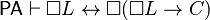$\mathsf{PA} \vdash \Box L \leftrightarrow \Box(\Box L \to C)$
2.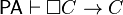$\mathsf{PA} \vdash \Box C \to C$
3.$\mathsf{PA} \vdash \Box(\Box L \to C) \to (\Box \Box L \to \Box C)$
4.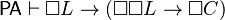$\mathsf{PA} \vdash \Box L \to (\Box \Box L \to \Box C)$
5.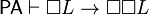$\mathsf{PA} \vdash \Box L \to \Box \Box L$
6.$\mathsf{PA} \vdash \Box L \to \Box C$
7.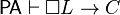$\mathsf{PA} \vdash \Box L \to C$
8.$\mathsf{PA} \vdash \Box(\Box L \to C)$
9.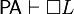$\mathsf{PA} \vdash \Box L$
10.$\mathsf{PA} \vdash C$